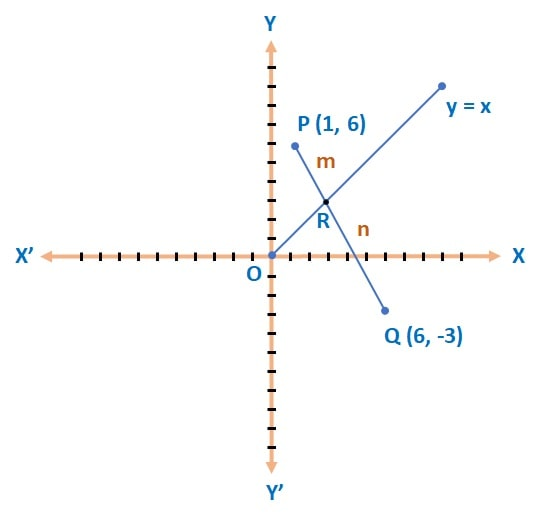Q) Find the ratio in which line y = x divides the line segment joining the points (6, -3) and (1, 6).

Ans: Let’s draw the diagram to solve:Also consider that the line y = x divided the line PQ in the ratio of m : n.

By section formula, if a point (x, y) divides the line joining the points (x1, y1​) and (x2​, y2​) in the ratio m : n, then coordinates of point R (x, y) =
(,)

Here,

P (1, 6) = (x1, y1​)

Q (6, -3) = (x2​, y2​)

Since the line PQ is divided in the ratio of m : n, Hence the co-ordinates of point P:

x =Similarly, y =Since the point R (x,y) lies on the line y = x, therefore it should satisfy the condition.∴ (-3 m + 6 n)  = (6 m + n)

9 m = 5 n

m : n = 5 : 9

Therefore, the line y = x divides the given line segment in the ratio of 5: 9.

Scroll to Top